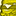# New to Qlik Sense

If you’re new to Qlik Sense, start with this Discussion Board and get up-to-speed quickly.

Announcements
Our May 2021 end-to-end product release from Data Integration to Data Analytics is out! READ DETAILS
cancel
Showing results for
Did you mean:Contributor II

## Why even use set analysis ?

What's the point of using set analysis since it accepts only Data Model Fields and not Dimensions .

1. I see that almost every case where we need set analysis, it can be replaced by a combination of other functions like aggr(), if() etc. So do we really require set analysis?

2. I have a date field in my data model called "order_date" and dimensions "order_year", "order_week" which are derived from "order_date" field using formula Year(order_date) and Week(order_date) . I can't use these two dimensions in my set expressions. For eg, I can't use these dimensions like this

=Sum({<order_year={"<=\$(=vMaxYear)"}>}Sales)

because "order_year" being a dimension can't be used and this formula will throw an error.   So how do you handle situations like these and does Set analysis even help when I have my Calendar fields as Dimensions? If so, in what cases?

@Henric_Cronström @sunny_talwar @swuehl

Labels (5)

• ### set expressions

1 Solution

Accepted SolutionsEmployee

No, you need set analysis.

1. You don't always get the same result using other functions. For example, one would think that

Sum( If(OrderMonth=4 and InvoiceMonth=5, Amount) )
Sum( {<OrderMonth={4}, InvoiceMonth={5}>} Amount) )

would result in the same number, but this is not necessarily true: The If()-function generates a join between the tables that contain OrderMonth, InvoiceMonth, and Amount, and if there is a many-to-many relationship, you may get duplicate records. I.e. the same dollar will be counted several times. I.e. an incorrect result.

The set analysis, on the other hand, does not cause a join. Here the numbers will be correct.

In addition, the set analysis evaluates faster if you have large data amounts.

2. Try
Sum({<order_date.autoCalendar.order_year={"<=\$(=vMaxYear)"}>} Sales)

HIC

5 RepliesEmployee

No, you need set analysis.

1. You don't always get the same result using other functions. For example, one would think that

Sum( If(OrderMonth=4 and InvoiceMonth=5, Amount) )
Sum( {<OrderMonth={4}, InvoiceMonth={5}>} Amount) )

would result in the same number, but this is not necessarily true: The If()-function generates a join between the tables that contain OrderMonth, InvoiceMonth, and Amount, and if there is a many-to-many relationship, you may get duplicate records. I.e. the same dollar will be counted several times. I.e. an incorrect result.

The set analysis, on the other hand, does not cause a join. Here the numbers will be correct.

In addition, the set analysis evaluates faster if you have large data amounts.

2. Try
Sum({<order_date.autoCalendar.order_year={"<=\$(=vMaxYear)"}>} Sales)

HICContributor II
Author

Thanks a lot for the explanation. It makes sense

If there are any articles/blogs that explains such things in detail please share. It would be very helpfulEmployee

There is a lot. It may be hard to find, though... Start with the following:

HICContributor II
Author

This formula works

YEAR is a field in the data model

Sum({1<YEAR={"\$(=\$(v_CurrentYear))"}>} Sales )

But this formula doesn't work

Sum({<order_date.autoCalendar.order_year={"\$(=\$(v_CurrentYear))"}>} Sales )

dimension order_year created using formula = num(year(DATE))

v_CurrentYear = num(Year(Today()))

Any help on why it is not working and how to deal with it?MVP

You can prepare your dimensions as fields in the data model, using a calendar script or the auto-generated Qlik Sense calendar fields Henric was referring to. If you don't want to, try something like

=Sum({<order_date = {"=year(order_date)<=\$(=vMaxYear)"}>} Sales)

(translates to something like: select the values in data model field order_date, where year(order_date) <= vMaxYear)